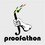# CMC - Problem 7

Problem 7. (3 points) The IPhOO has an ongoing Facebook competition where anyone at any time can answer with a chance to win points. A correct answer to a problem gets the full number of points the problem offers. The first 6 problems were worth the following number of points each:

1. 1 point
2. 3 points
3. 4 points
4. 7 points
5. 9 points
6. 3 points (note that this is an inaccurate statistic in real life, but assume it to be true for this problem)

Assuming people are indistinguishable, how many different possible outcomes are there? Note that an outcome is a set of people each having a positive number of points.Note by Cody Johnson
6 years, 7 months ago

This discussion board is a place to discuss our Daily Challenges and the math and science related to those challenges. Explanations are more than just a solution — they should explain the steps and thinking strategies that you used to obtain the solution. Comments should further the discussion of math and science.

When posting on Brilliant:

• Use the emojis to react to an explanation, whether you're congratulating a job well done , or just really confused .
• Ask specific questions about the challenge or the steps in somebody's explanation. Well-posed questions can add a lot to the discussion, but posting "I don't understand!" doesn't help anyone.
• Try to contribute something new to the discussion, whether it is an extension, generalization or other idea related to the challenge.

MarkdownAppears as
*italics* or _italics_ italics
**bold** or __bold__ bold
- bulleted- list
• bulleted
• list
1. numbered2. list
1. numbered
2. list
Note: you must add a full line of space before and after lists for them to show up correctly
paragraph 1paragraph 2

paragraph 1

paragraph 2

[example link](https://brilliant.org)example link
> This is a quote
This is a quote
    # I indented these lines
# 4 spaces, and now they show
# up as a code block.

print "hello world"
# I indented these lines
# 4 spaces, and now they show
# up as a code block.

print "hello world"
MathAppears as
Remember to wrap math in $$ ... $$ or $ ... $ to ensure proper formatting.
2 \times 3 $2 \times 3$
2^{34} $2^{34}$
a_{i-1} $a_{i-1}$
\frac{2}{3} $\frac{2}{3}$
\sqrt{2} $\sqrt{2}$
\sum_{i=1}^3 $\sum_{i=1}^3$
\sin \theta $\sin \theta$
\boxed{123} $\boxed{123}$

Sort by:

"The first 5 problems" should be "the first 6 problems?"

- 6 years, 7 months ago

How do you expect me to know how to count???

- 6 years, 7 months ago

- 6 years, 7 months ago

Is having a score of zero not included?

- 6 years, 7 months ago

Every problem was solved. If you didn't solve any, then you're not included in the results.

- 6 years, 7 months ago

I mean in the problem... in the contest, is it possible for a person to have a score of zero?

- 6 years, 7 months ago

See that each person must have a positive number of points.

- 6 years, 7 months ago

That's what I was talking about. Every problem was solved in the IPhOO Facebook contest was solved. If you didn't solve any IPhOO Facebook contest problems, then you're not included in the results.

- 6 years, 7 months ago

25

- 6 years, 7 months ago

The correct answer was 98. Whoever posts their solution will get the full 3 points.

- 6 years, 7 months ago

If you also include the different questions answered as part of the calculation , then you should mention that in the question.

All I understood from the question was that only the final marks is to be considered irrespective of whether one can get that by answering two questions or get the same by answering one ...

- 6 years, 7 months ago